PRINTABLE FOR KIDS

XII (12) HSC

XI (11) FYJC
X (10) SSC

### Practice Set 5.3 Co-ordinate Geometry Class 10th Mathematics Part 2 MHB Solution

Practice Set 5.3

1. Angles made by the line with the positive direction of X-axis are given. Find the slope…
2. Find the slopes of the lines passing through the given points. (1) A (2, 3), B (4, 7)…
3. Determine whether the following points are collinear. (1) A(-1, -1), B(0, 1), C(1, 3)…
4. If A (1, -1),B (0, 4),C (-5, 3) are vertices of a triangle then find the slope of each…
5. Show that A (-4, -7),B (-1, 2), C (8, 5) and D (5, -4) are the vertices of a…
6. Find k, if R(1, -1), S (-2, k) and slope of line RS is -2.
7. Find k, if B(k, -5), C (1, 2) and slope of the line is 7.
8. Find k, if PQ || RS and P(2, 4), Q (3, 6), R(3, 1), S(5, k) .

###### Practice Set 5.3
Question 1.

Angles made by the line with the positive direction of X-axis are given. Find the slope of these lines.

(1) 45°

(2) 60°

(3) 90°

slope is given as the tangent of the angle formed with the positive direction of x-axis

1. tan 45° = 1

2. tan60° =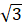3. tan 90° = cannot be determined.

Question 2.

Find the slopes of the lines passing through the given points.
(1) A (2, 3), B (4, 7)

(2) P (-3, 1), Q (5, -2)

(3) C (5, -2), D (7, 3)

(4) L (-2, -3), M (-6, -8)

(5) E(-4, -2), F (6, 3)

(6) T (0, -3), S (0, 4)

Slope m of a line passing through two points A(a,b) and B(c,d) ig given by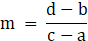1. A (2, 3), B (4, 7)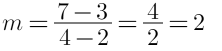2. P (-3, 1), Q (5, -2)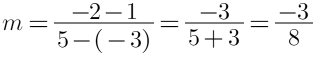3. C (5, -2), D (7, 3)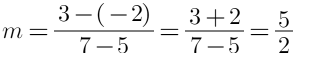4. L (-2, -3), M (-6, -8)5. E(-4, -2), F (6, 3)6. T (0, -3), S (0, 4)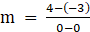As denominator is 0,

So, slope cannot be determined.

Question 3.

Determine whether the following points are collinear.
(1) A(-1, -1), B(0, 1), C(1, 3)

(2) D(-2, -3), E(1, 0), F(2, 1)

(3) L(2, 5), M(3, 3), N(5, 1)

(4) P(2, -5), Q(1, -3), R(-2, 3)

(5) R(1, -4), S(-2, 2), T(-3, 4)

(6) A(-4, 4), K(-2, 5/ 2 ), N(4, -2)

Three points are said to be collinear if they all lie in a straight line.
If Three points (x1, y1), (x2y2), (x3y3) are collinear then no triangle can be formed using three points and so the area formed by the triangle by the three points is zero.

Area of Triangle = 1/2 [x(y− y3) + x(y− y1) + x(y− y2)] ...(1)

1. For triangle, A(-1, -1), B(0, 1), C(1, 3)
Area =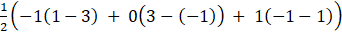= 1/2 [ -1(-2) + 0(3+1) + 1(-1-1)]

= 1/2 [2 + 0 - 2]

= 1/2 [2-2]
= 0
Hence the points are collinear

2. For triangle, D(-2, -3), E(1, 0), F(2, 1)
Using 1,
Area = 1/2 [-2(0-1) + 1(1-(-3)) + 2(-3-0)]
= 1/2 [-2(-1) + 1(1+3) + 2(-3)]
= 1/2 [ 2 + 4 -6 ]
= 1/2 [ 6 - 6 ]
= 1/2 (0)
= 0
Hence the points are collinear.

3. For triangle, L(2, 5), M(3, 3), N(5, 1)
Using 1,

Area = 1/2 [2(3-1) + 3(1-5) + 5(5-3)]
= 1/2 [ 2(2) + 3(-4) + 5(2)]
= 1/2 [ 4 - 12 + 10 ]
= 1/2 [ 14 - 12 ]

= 1/2 (2)
= 1

Hence the points are not collinear.

4. For triangle, P(2, -5), Q(1, -3), R(-2, 3)
Using 1,
Area =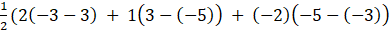= 0
Area = 1/2 [ 2(-3-3) + 1(3-(-5)) + (-2)(-5-(-3))]
= 1/2 [ 2(-6) + 1(3+5) - 2 (-5+3)]
= 1/2 [ -12 + 8 -2(-2)]
= 1/2 [-12 + 8 +4]
= 1/2 [ -12 + 12]
= 1/2 (0)

= 0

Hence the points are collinear.

5. For triangle, R(1, -4), S(-2, 2), T(-3, 4)
Area =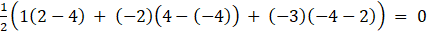Hence the points are collinear.

6. For triangle, A(-4, 4), K(-2, 5/ 2 ), N(4, -2)
Area =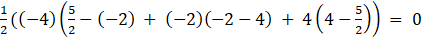Hence the points are collinear.

Question 4.

If A (1, -1),B (0, 4),C (-5, 3) are vertices of a triangle then find the slope of each side

Slope m of a line passing through two points A(a,b) and B(c,d) is given bySlope of AB =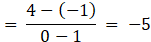Slope of BC =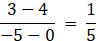Slope of AC =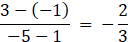Question 5.

Show that A (-4, -7),B (-1, 2), C (8, 5) and D (5, -4) are the vertices of a parallelogram.

In a parallelogram, opposite sides are equal and parallel.

According to the distance formula, the distance 'd' between two points (a,b) and (c,d) is given by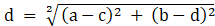.....(1)

Slope m of a line passing through two points A(a,b) and B(c,d) ig given byIn the question,

AB =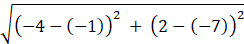=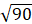BC =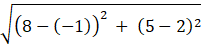=CD =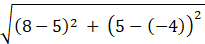=AD =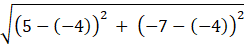=Slope of AB =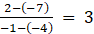Slope of BC =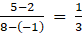Slope of CD =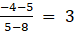Slope of AD =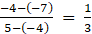As AB = DC and BC = AD

And Slope AB = Slope CD

Slope BC = slope AD

Hence the given points form a parallelogram.

Question 6.

Find k, if R(1, -1), S (-2, k) and slope of line RS is -2.

Slope m of a line passing through two points A(a,b) and B(c,d) ig given byIn the given question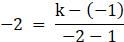Simplifying

6 = k + 1

K = 5

Question 7.

Find k, if B(k, -5), C (1, 2) and slope of the line is 7.

Slope m of a line passing through two points A(a,b) and B(c,d) ig given byIn the given question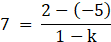Simplifying

7-7k = 7

k = 0

Question 8.

Find k, if PQ || RS and P(2, 4), Q (3, 6), R(3, 1), S(5, k) .

two lines are said to be parallel if their slopes are equal

If PQ||RS then their slopes must be equal

Slope of PQ =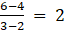Slope pf RS =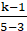As their slopes are equal, we get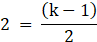Simplifying

k-1 = 4

k = 5

## PDF FILE TO YOUR EMAIL IMMEDIATELY PURCHASE NOTES & PAPER SOLUTION. @ Rs. 50/- each (GST extra)

SUBJECTS

HINDI ENTIRE PAPER SOLUTION

MARATHI PAPER SOLUTION
SSC MATHS I PAPER SOLUTION
SSC MATHS II PAPER SOLUTION
SSC SCIENCE I PAPER SOLUTION
SSC SCIENCE II PAPER SOLUTION
SSC ENGLISH PAPER SOLUTION
SSC & HSC ENGLISH WRITING SKILL
HSC ACCOUNTS NOTES
HSC OCM NOTES
HSC ECONOMICS NOTES
HSC SECRETARIAL PRACTICE NOTES

2019 Board Paper Solution

HSC ENGLISH SET A 2019 21st February, 2019

HSC ENGLISH SET B 2019 21st February, 2019

HSC ENGLISH SET C 2019 21st February, 2019

HSC ENGLISH SET D 2019 21st February, 2019

SECRETARIAL PRACTICE (S.P) 2019 25th February, 2019

HSC XII PHYSICS 2019 25th February, 2019

CHEMISTRY XII HSC SOLUTION 27th, February, 2019

OCM PAPER SOLUTION 2019 27th, February, 2019

HSC MATHS PAPER SOLUTION COMMERCE, 2nd March, 2019

HSC MATHS PAPER SOLUTION SCIENCE 2nd, March, 2019

SSC ENGLISH STD 10 5TH MARCH, 2019.

HSC XII ACCOUNTS 2019 6th March, 2019

HSC XII BIOLOGY 2019 6TH March, 2019

HSC XII ECONOMICS 9Th March 2019

SSC Maths I March 2019 Solution 10th Standard11th, March, 2019

SSC MATHS II MARCH 2019 SOLUTION 10TH STD.13th March, 2019

SSC SCIENCE I MARCH 2019 SOLUTION 10TH STD. 15th March, 2019.

SSC SCIENCE II MARCH 2019 SOLUTION 10TH STD. 18th March, 2019.

SSC SOCIAL SCIENCE I MARCH 2019 SOLUTION20th March, 2019

SSC SOCIAL SCIENCE II MARCH 2019 SOLUTION, 22nd March, 2019

XII CBSE - BOARD - MARCH - 2019 ENGLISH - QP + SOLUTIONS, 2nd March, 2019

HSC Maharashtra Board Papers 2020

(Std 12th English Medium)

HSC ECONOMICS MARCH 2020

HSC OCM MARCH 2020

HSC ACCOUNTS MARCH 2020

HSC S.P. MARCH 2020

HSC ENGLISH MARCH 2020

HSC HINDI MARCH 2020

HSC MARATHI MARCH 2020

HSC MATHS MARCH 2020

SSC Maharashtra Board Papers 2020

(Std 10th English Medium)

English MARCH 2020

HindI MARCH 2020

Hindi (Composite) MARCH 2020

Marathi MARCH 2020

Mathematics (Paper 1) MARCH 2020

Mathematics (Paper 2) MARCH 2020

Sanskrit MARCH 2020

Important-formula

THANKS